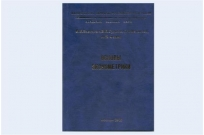Center for Strategic Assessment and forecasts

Autonomous non-profit organization

Home / Science and Society / Analytical work: the experience of Russian and foreign experts / Recommended
Baskakov V. V., Gudkov, B. N. , Fedoseev S. A. ,Fomin A. N. "Fundamentals of econometrics (Course for military systems engineers)"Material posted: Publication date: 26-02-2013

The monograph presents the methodological foundations of econometrics, including the required mathematical apparatus of probability theory and mathematical statistics, the theory of the steam room and multiple regression, theory of systems of econometric equations, the theory of one-dimensional time series and its application to predict dynamics of complex systems.

The emphasis is on generic methods and techniques, have different economic systems.

For clarity of presentation of theoretical material supplemented with specific numerical examples algorithms for solving various practical problems. They allow readers to understand the technology of econometric studies.

At the end of the monograph deals with theoretical questions related to the theorem Gauss-Markov, and with one version of a mathematical model of world dynamics.

In addition, the monograph presents the key terms in the subject area.

The monograph can be of interest for analysts, systems engineers, specialists on mathematical modeling and strategic planning, managers, researchers, teachers, postgraduates and students.

Vladimir BASKAKOV, B. N. GUDKOV, S. A. FEDOSEEV, A. N. FOMIN

"Fundamentals of econometrics (Course for military systems engineers)": Monograph. M.: VA strategic missile forces. Peter The Great, 2013.

***

Econometrics is the science that studies quantitative and qualitative economic relationships using mathematical and statistical methods and models. Its main goal is to use statistics and mathematics to economic theory.

Econometrics allows to statistically evaluate the validity of mathematical models from the point of view of their adequacy to have the amount of source data. In order to vividly highlight the importance of econometrics to the economy, it is no exaggeration to say that without the "permission" of econometrics economic models cannot be applied to situation analysis and forecasting.

Econometrics provides the tools for economic measurement and the methodology for estimation of parameters of models of micro - and macroeconomics.

The term "econometrics" consists of two parts: the "economy" from "Economics" and "metric" "measurement". Econometrics is included in the broad family of disciplines devoted to the measurement and application of statistical methods in various fields of science and practice. To this family belong, in particular, biometrics, technometrics, scientometrics, psychometry, qualimetry.

For the development of econometrics proposed structure of the monograph.

The first part describes the theoretical minimum required for subsequent solution of practical problems. Will be considered the basic tenets of the theory of probabilities as applied to econometrics, the student distribution and the Fisher, the theoretical basis of the method of least squares, methods for solving systems of linear equations, bases of the theory of statistical hypothesis testing, numerical methods of optimization of functions of one and several variables.

Below will be considered specific examples of econometric problems steam room and multiple regression. This part will conclude with a theoretical consideration of the General case of multiple linear regression, with which readers will face when solving practical problems.

Next, we will move from consideration of individual regression equations to systems of linear equations. It turns out that it is not so simple – to connect the individual equations in the system. There are some limitations, some of which can be overcome using a modified method of least squares.

Then we will deal with time series, i.e. sets of effective parameters that are linked to specific moments in time. Will be considered additive and multiplicative models of time series and raised very complex issues of forecasting their future values. Illustrate the application of moving averages time series will be presented in relation to technical analysis of financial markets.

Finally, the last Chapter in the enacted plan will address the problems of drafting and sizing equations global dynamics, which are studied by the club of Rome. This will be done to make readers more clearly understand the complexity of the methodological problems faced by Economics in General and econometrics in particular.

It should be noted that, as often happens in science, the techniques of econometrics, which was originally established for economic research proved to be more versatile. They with success can be applied in other areas that require elaboration of mathematical models with a small amount of initial information.

RELATED MATERIALS: Science and Society